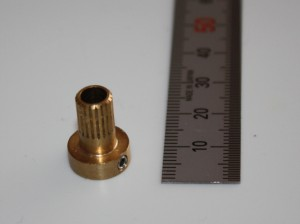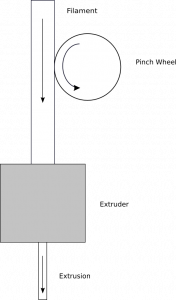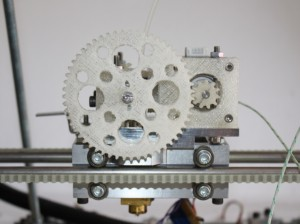## Calculating E_STEPS_PER_MM for the Reprap Mendel

UPDATE: Nophead now covers this better in this post.

In the course of building my extruder I chose not to modify my stepper motor. Instead of having a splined shaft on the motor I decided to use a splined pinch wheel similar to the brass M4 insert used in the geared extruder driver. My pinch wheel is shown below in Figure 1. By making some simple assumptions and using basic geometry I was able to calculate the necessary firmware parameter to accommodate my changes from the original design.Figure 1: Splined pinch wheel. It is designed to fit a shaft with a diameter of 5mm and is fixed in place using a M3x3 grub screw.

I wanted to keep the motor unmodified so that it would be interchangeable with any of the other motors I’ve used on the reprap. The upside of this is that I don’t need to keep a separate modified motor as a spare just for the extruder. The downside of this is that the default value for `E_STEPS_PER_MM` used in the reprap motherboard firmware would not be correct for my extruder.

#### What does E_STEPS_PER_MM do?

`E_STEPS_PER_MM` is defined in the firmware documentation as “the number of steps that the extruder stepper needs to take to produce one millimetre of filament.” The firmware then uses this value to calculate the number of steps needed to drive the extruder in relation the print head’s current horizontal movement. If this value is wrong then the reprap will push out too much or too little extrusion into the print.

In the absence of any commissioning information in the official wiki for the reprap, once construction of the reprap was complete, I was left to adjust the value empirically.

As I had no way of knowing just how different my untested design would be to whatever extruder was used to determine the values given in the code, I wanted another way to determine this value. These calculations are used as a check to avoid wasting plastic and the possibility of breaking the extruder first time.

To simplify things I chose to calculate the parameter by treating the filament and melted plastic as an incompressible fluid. This simplification should hold true once the system is in a steady state extruding plastic. If no material leaks out, is absorbed into the body of the extruder itself, or is vaporized, then logically the volume of material that goes into the system must also leave the system. This also assumes that there is no thermal expansion or any change in density of the material (which is what I really mean when I say “incompressible”). Figure 2 below shows a diagram of my greatly simplified model of the system.Figure 2: Model of the simplified system shown with the extrusion chamber shown as a grey box.

Bear in mind that I’m not a mechanical or chemical engineer. I’m an electrical engineer, so my calculations and assumptions might be way off. If anyone knows a better way to model and or calculate this sort of thing, I’d love to hear from you.

### What goes in, must come out

#### Calculating the volume of filament entering the extruder:

The volume that goes into the extruder is the cross sectional area of filament multiplied by the length fed in. This assumes the filament is a perfect cylinder.
``` rf = 0.5 × Øf (filament diameter) CSAf(filament cross sectional area) = π × rf² volf (filament volume per revolution) = CSAf × lenf ```

Assuming the filament is moving at the same speed as the outermost point on the teeth of the drive gear or surface of the pinch wheel then the length of filament fed in should be the same as the linear distance travelled by the outside of the pinch wheel. This is assuming that no slippage, no compression, and no deformation of the filament occur.

A point on the outside of the pinch wheel travels a linear distance of one circumference per revolution. This is calculated by π multiplied by the diameter.
``` ØPW (pinch wheel diameter) lenf (filament length per revolution) = π × ØPW ```

The total volume entering the extruder per step is easily calculated by dividing the volume per revolution by the number of steps per revolution. If a gear reduction is used between the stepper and then the steps per revolution are multiplied by this ratio.
``` RM:PW (ratio of motor rpm to pinch wheel rpm) steps360 (motor steps per revolution)```

``` step-volf (filament volume per step) = volf steps360 × RM:PW ```

#### Real world examples

In addition to the standard extruder I have also built a version of Adrian’s geared extruder. My version of this is shown in Figure 3 below. The ratio for this gear reduction is 5:1. I will compare this with the standard splined shaft extruder in the calculations below.Figure 3: A geared extruder. The large gear has 55 teeth and the small gear has 11 giving a 5:1 reduction.

Using filament with a nominal diameter of 3mm gives:
``` rf = 0.5 × Øf = 1.5mm CSAf = π × rf² = 7.07mm² ```

For a standard reprap using a 5mm diameter splined shaft:
``` ØPW = 5.0 mm lenf = π × ØPW = 15.7mm volf = CSAf × lenf = 111mm³ ```

With a 200 steps per revolution stepper motor, driven using half steps and directly driving the pinch wheel:
``` RM:PW = 1```

``` step-volf = 111mm³ 400 × 1 ```

```step-volf = 0.278mm³ ```

Alternatively, with the same motor driven using half steps but driving via a 5:1 gear reduction and a 8mm brass insert:
``` ØPW = 8.0 mm lenf = π × ØPW = 25.1mm volf = CSAf × lenf = 177mm³ RM:PW = 5```

``` step-volf = 177mm³ 400 × 5 ```

```step-volf = 0.0885mm³ ```

This means that for each half step of the motor the original extruder draws in 0.278mm³ of plastic. If the geared extruder is used then the volume of plastic drawn in with each step of the motor is an order of magnitude lower at 0.0885mm³. This means that the geared extruder should have much finer control as well as increased torque.

#### Calculating the length of extrusion leaving the extruder:

The output of the system is via a smaller diameter nozzle. Working from our main assumption, what goes in must come out, the system must extrude all of the material entering the system but with a smaller cross sectional area.

Assuming the extrusion is the same as the nozzle diameter:
``` Øe (extrusion diameter) = ØNOZZLE re (extrusion radius) = 0.5 × Øe CSAe (cross sectional area of extrusion) = π × re² ```
Reworking our formulas from above gives us the length from a known volume. This assumes the extrusion, like the filament, is also a perfect cylinder.
``` lene (length of extrusion) vole (extrusion volume ) = lene × CSAe ```

``` lene = vole CSAe ```

Substituting in the known volume drawn into the system per step:
``` vole = step-volf ```

``` lene = step-volf CSAe ```

This is the length of extrusion output per step. By inverting this we get the number of steps per mm of extrusion required by the software.

``` E_STEPS_PER_MM = CSAe step-volf ```

#### Real world examples (continued from above)

A nozzle with a 0.5mm hole and using a splined 5mm shaft direct drive system from above:
``` Øe = 0.5mm step-volf = 0.278mm³ (from earlier example above) re = 0.5 × Øe = 0.25mm CSAe = π × re² = 0.196mm²```

``` E_STEPS_PER_MM = 0.196mm² 0.278mm³ ```

```E_STEPS_PER_MM = 0.705 ```

A nozzle with a 0.5mm hole and using a 5:1 gear reduction and a 8mm brass insert from above:
``` Øe = 0.5mm step-volf = 0.0885mm³ (from earlier example above) re = 0.5 × Øe = 0.25mm CSAe = π × re² = 0.196mm²```

``` E_STEPS_PER_MM = 0.196mm² 0.0885mm³ ```

```E_STEPS_PER_MM = 2.21 ```

These values match up well with the values for the standard extruders given in the firmware.

### Tying it all together

After reading through the working above you can see the result is proportional to the ratio of the square of the diameters and some of the terms will cancel out.

Combining and simplifying gives a single formula:

``` E_STEPS_PER_MM = ØNOZZLE² × steps360 × RM:PW Øf² × π × ØPW ```

Where:

``` Øf =  filament diameter ØPW =  pinch wheel diameter ØNOZZLE =  nozzle diameter steps360 =  number of steps per revolution RM:PW =  ratio of motor revolutions to pinch wheel revolutions ```

Below in Listing 1 are my changes to `configuration.h` to automatically calculate the steps per mm based on the characteristics of the extruder. This is calculated when the main firmware is compiled. I’ve also created a google spreadsheet here to help calculate the same value if you just want the number to throw straight into `configuration.h`. These values form a good starting point for the inevitable fine-tuning which is required later.

Any thoughts, comments and ideas are always welcome.